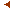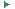this issueprevious article in this issue next article in this issueDocument Details : Title: On the Analysis of the Truncated Generalized Poisson Distribution Using a Bayesian Method Author(s): SCOLLNIK, D.P.M. Journal: ASTIN Bulletin Volume: 28    Issue: 1   Date: May 1998   Pages: 135-152 DOI: 10.2143/AST.28.1.519083 Abstract : The generalized Poisson distribution with parameters theta and lambda was introduced by Consul and Jain (1973) and has recently found several instances of application in the actuarial literature. The most frequently used version of the distribution assumes that theta > 0 and 0 < lambda < 1, in which case the mean and variance are theta / ( 1 - lambda) and theta / ( 1 - lambda)^3, respectively. These simple moment expressions, along with nearly all of the other theoretical results available for this distribution, fail when lambda < 0 or lambda >1 (e.g., Johnson, Kotz, and Kemp, 1992, page 397). In these cases, even the definition of the probability mass function usually given in the literature as not properly normalized so that its values do not sum to unity. For this reason, it is common to truncate the support of the distribution and explicitly normalize the probability mass function. In this paper we discuss the estimation of the parameters of this truncated generalized Poisson distribution using a Bayesian method

18.204.2.231.